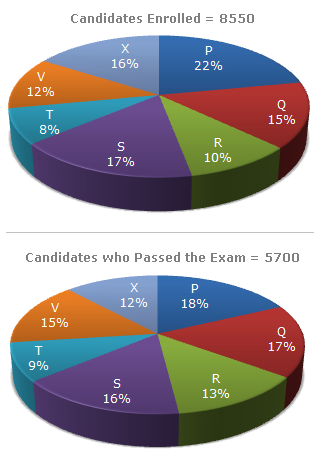# Data Interpretation - Pie Charts

### Exercise :: Pie Charts - Pie Chart 10

Study the following graph carefully and answer the questions given below:

Distribution of candidates who were enrolled for MBA entrance exam and the candidates (out of those enrolled) who passed the exam in different institutes:1.

What percentage of candidates passed the Exam from institute T out of the total number of candidates enrolled from the same institute?

 A. 50% B. 62.5% C. 75% D. 80%

Explanation:

 Required percentage =9% of 5700 x 100% =9 x 5700 x 100% = 75%. 8% of 8550 8% of 8550

2.

Which institute has the highest percentage of candidates passed to the candidates enrolled?

 A. Q B. R C. V D. T

Explanation:

The percentage of candidates passed to candidates enrolled can be determined for each institute as under:

 (i) P =18% of 5700x 100% =18 x 5700 x 100% = 54.55%. 22% of 8550 22 x 8550

 (ii) Q =17% of 5700x 100% = 75.56%. 15% of 8550

 (iii) R =13% of 5700x 100% = 86.67%. 10% of 8550

 (iv) S =16% of 5700x 100% = 62.75%. 17% of 8550

 (v) T =9% of 5700x 100% = 75%. 8% of 8550

 (vi) V =15% of 5700x 100% = 83.33%. 12% of 8550

 (vii) X =12% of 5700x 100% = 50%. 16% of 8550

Highest of these is 86.67% corresponding to institute R.

3.

The number of candidates passed from institutes S and P together exceeds the number of candidates enrolled from institutes T and R together by:

 A. 228 B. 279 C. 399 D. 407

Explanation:

 Required difference = [(16% + 18%) of 5700] - [(8% + 10%) of 8550] = [(34% of 5700) - (18% of 8550)] = (1938 - 1539) = 399.

4.

What is the percentage of candidates passed to the candidates enrolled for institutes Q and R together?

 A. 68% B. 80% C. 74% D. 65%

Explanation:

 Candidates passed from institutes Q and R together = [(13% + 17%) of 5700] = 30% of 5700. Candidates enrolled from institutes Q and R together = [(15% + 10%) of 8550] = 25% of 8550.Required Percentage
 =30% of 5700 x 100% 25% of 8550
 =30 x 5700 x 100% 25 x 8550
= 80%.

5.

What is the ratio of candidates passed to the candidates enrolled from institute P?

 A. 9 : 11 B. 14 : 17 C. 6 : 11 D. 9 : 17

 Required ratio =18% of 5700=18 x 5700= 6 . 22% of 8550 22 x 8550 11